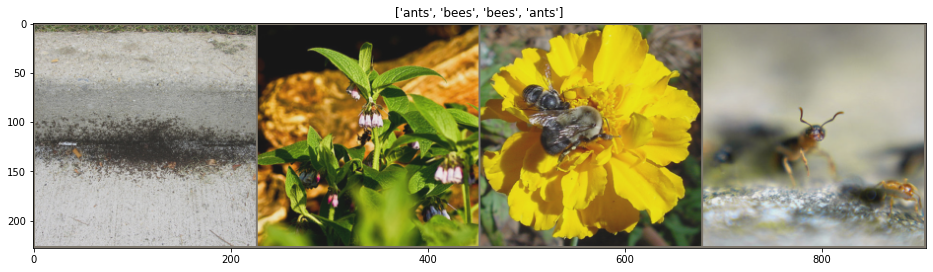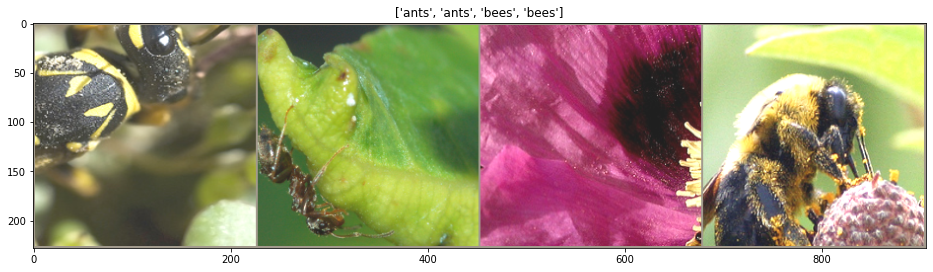# Transfer Learning for Computer Vision Tutorial¶

Author: Sasank Chilamkurthy <https://chsasank.github.io>_

In this tutorial, you will learn how to train a convolutional neural network for image classification using transfer learning. You can read more about the transfer learning at cs231n notes <https://cs231n.github.io/transfer-learning/>__

Quoting these notes,

In practice, very few people train an entire Convolutional Network
from scratch (with random initialization), because it is relatively
rare to have a dataset of sufficient size. Instead, it is common to
pretrain a ConvNet on a very large dataset (e.g. ImageNet, which
contains 1.2 million images with 1000 categories), and then use the
ConvNet either as an initialization or a fixed feature extractor for



These two major transfer learning scenarios look as follows:

• Finetuning the convnet: Instead of random initializaion, we initialize the network with a pretrained network, like the one that is trained on imagenet 1000 dataset. Rest of the training looks as usual.
• ConvNet as fixed feature extractor: Here, we will freeze the weights for all of the network except that of the final fully connected layer. This last fully connected layer is replaced with a new one with random weights and only this layer is trained.
In :
%matplotlib inline

In :
# License: BSD
# Author: Sasank Chilamkurthy

from __future__ import print_function, division

import torch
import torch.nn as nn
import torch.optim as optim
from torch.optim import lr_scheduler
import numpy as np
import torchvision
from torchvision import datasets, models, transforms
import matplotlib.pyplot as plt
import time
import os
import copy

plt.ion()   # interactive mode


The problem we're going to solve today is to train a model to classify ants and bees. We have about 120 training images each for ants and bees. There are 75 validation images for each class. Usually, this is a very small dataset to generalize upon, if trained from scratch. Since we are using transfer learning, we should be able to generalize reasonably well.

This dataset is a very small subset of imagenet.

.. Note :: Download the data from here <https://download.pytorch.org/tutorial/hymenoptera_data.zip>_ and extract it to the current directory.

In [ ]:
# dowload the data

!rm -rf hymenoptera_data*
!unzip hymenoptera_data.zip

In :
# Data augmentation and normalization for training
# Just normalization for validation

normalize_mean = [0.485, 0.456, 0.406]
normalize_std = [0.229, 0.224, 0.225]

data_transforms = {
'train': transforms.Compose([
transforms.RandomResizedCrop(224),
transforms.RandomHorizontalFlip(),
transforms.ToTensor(),
transforms.Normalize(normalize_mean, normalize_std)
]),
'val': transforms.Compose([
transforms.Resize(256),
transforms.CenterCrop(224),
transforms.ToTensor(),
transforms.Normalize(normalize_mean, normalize_std)
]),
}

data_dir = 'hymenoptera_data'
image_datasets = {x: datasets.ImageFolder(os.path.join(data_dir, x),
data_transforms[x])
for x in ['train', 'val']}
shuffle=True, num_workers=4)
for x in ['train', 'val']}
dataset_sizes = {x: len(image_datasets[x]) for x in ['train', 'val']}
class_names = image_datasets['train'].classes

device = torch.device("cuda:0" if torch.cuda.is_available() else "cpu")


Visualize a few images ^^^^^^^^^^^^^^^^^^^^^^ Let's visualize a few test and training images so as to understand the data augmentations.

In :
def imshow(inp, title=None, rescale=True):
"""Imshow for Tensor."""
if len(inp.shape)==2:
inp = np.tile(inp[None,:,:],(3,1,1))
if rescale:
inp = (inp - np.mean(inp)) / (4*np.std(inp)) + .5
inp = inp.transpose((1, 2, 0))
inp = np.clip(inp, 0, 1)
plt.imshow(inp)
if title is not None:
plt.title(title)

# Display a row of teest data and a row of train data
for data_type in 'val', 'train':
inputs = torchvision.utils.make_grid(inputs)
inputs = inputs.numpy() * np.array(normalize_std)[:,None,None] + np.array(normalize_mean)[:,None,None]
print(data_type,'data:')
plt.figure(figsize=(16,8))
imshow(inputs, title=[class_names[x] for x in classes])
plt.show()

val data:train data:## Training the model¶

Now, let's write a general function to train a model. Here, we will illustrate:

• Scheduling the learning rate
• Saving the best model

In the following, parameter scheduler is an LR scheduler object from torch.optim.lr_scheduler.

In :
def train_model(model, criterion, optimizer, scheduler, num_epochs=25):
since = time.time()

best_model_wts = copy.deepcopy(model.state_dict())
best_acc = 0.0

for epoch in range(num_epochs):
print('Epoch {}/{}'.format(epoch, num_epochs - 1))
print('-' * 10)

# Each epoch has a training and validation phase
for phase in ['train', 'val']:
if phase == 'train':
model.train()  # Set model to training mode
else:
model.eval()   # Set model to evaluate mode

running_loss = 0.0
running_corrects = 0

# Iterate over data.
inputs = inputs.to(device)
labels = labels.to(device)

# forward
# track history if only in train
outputs = model(inputs)
_, preds = torch.max(outputs, 1)
loss = criterion(outputs, labels)

# backward + optimize only if in training phase
if phase == 'train':
loss.backward()
optimizer.step()

# statistics
running_loss += loss.item() * inputs.size(0)
running_corrects += torch.sum(preds == labels.data)
if phase == 'train':
scheduler.step()

epoch_loss = running_loss / dataset_sizes[phase]
epoch_acc = running_corrects.double() / dataset_sizes[phase]

print('{} Loss: {:.4f} Acc: {:.4f}'.format(
phase, epoch_loss